Comparing Fractions – 4 Worksheets

Fraction

Fraction Circles

Fraction Circles Template

Fraction Model

Fraction Subtraction

Fractions – Coloring

Fractions – Comparing

Fractions – Equivalent

Fractions – Halves

Greater than, less than or equal to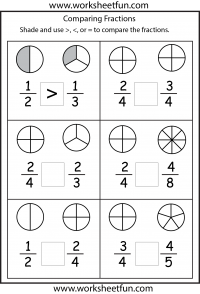greater than, less than or equal togreater than, less than or equal to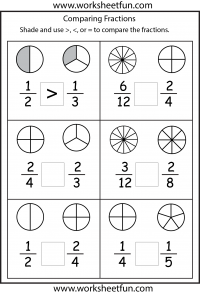greater than, less than or equal to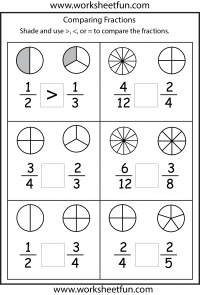greater than, less than or equal to

Fraction

Fraction Circles

Fraction Circles Template

Fraction Model

Fraction Subtraction

Fractions – Coloring

Fractions – Comparing

Fractions – Equivalent

Fractions – Halves

### New Worksheets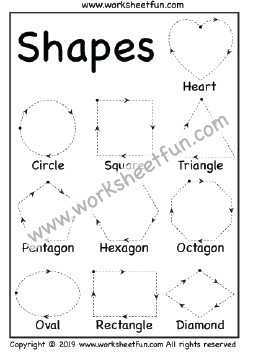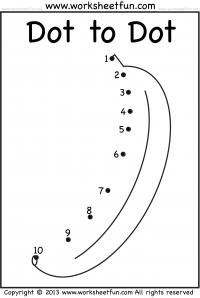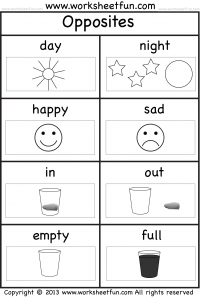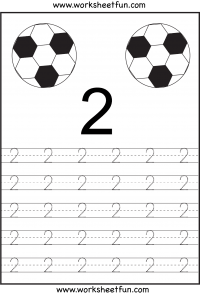### Most Popular Preschool and Kindergarten Worksheets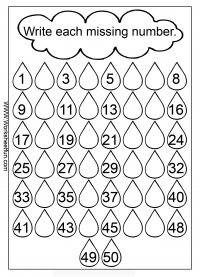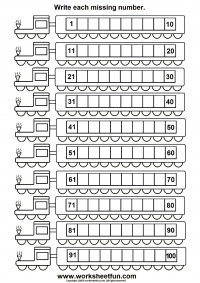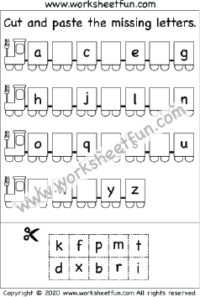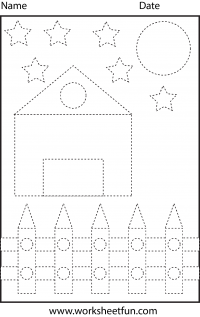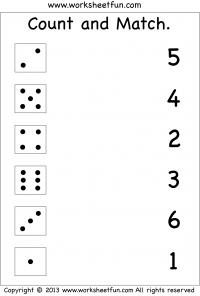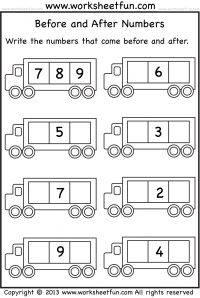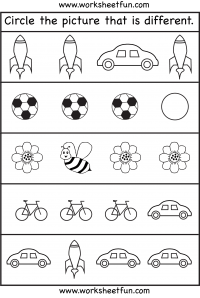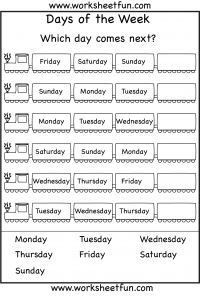#### Kindergarten Worksheets